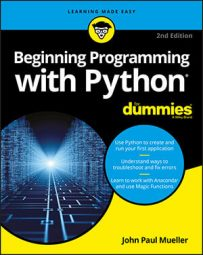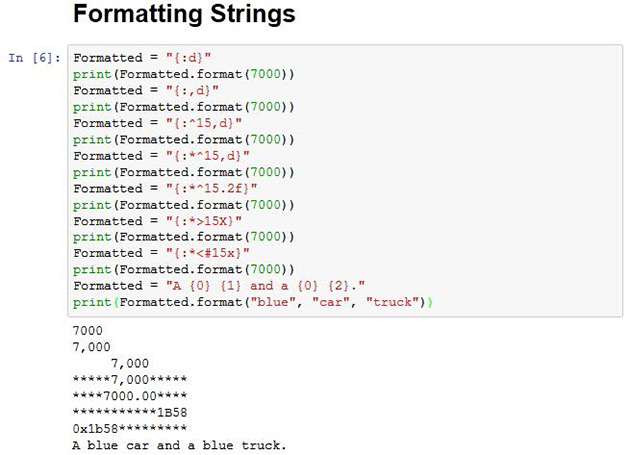##### Beginning Programming with Python For DummiesYou can format strings in a number of ways using Python. The main emphasis of formatting is to present the string in a form that is both pleasing to the user and easy to understand. Formatting doesn’t mean adding effects in this case, but refers merely to the presentation of the data. For example, the user might want a fixed-point number rather than a decimal number as output.

You have quite a few ways to format strings. However, the focus of most formatting is the format() function. You create a formatting specification as part of the string and then use the format() function to add data to that string. A format specification may be as simple as two curly brackets {} that specify a placeholder for data.

You can number the placeholder to create special effects. For example, {0} would contain the first data element in a string. When the data elements are numbered, you can even repeat them so that the same data appears more than once in the string.

The formatting specification follows a colon. When you want to create just a formatting specification, the curly brackets contain just the colon and whatever formatting you want to use.

For example, {:f} would create a fixed-point number as output. If you want to number the entries, the number that precedes the colon: {0:f} creates a fixed-point number output for data element one. The formatting specification follows this form, with the italicized elements serving as placeholders here:
`[[fill]align][sign][#][width][,][.precision][type]`
This specification provides you with the in-depth details, but here’s an overview of what the various entries mean:
• fill: Defines the fill character used when displaying data that is too small to fit within the assigned space.

• align: Specifies the alignment of data within the display space. You can use these alignments:

• <: Left aligned

• >: Right aligned

• ^: Centered

• =: Justified

• sign: Determines the use of signs for the output:

• +: Positive numbers have a plus sign and negative numbers have a minus sign.

• -: Negative numbers have a minus sign.

• <space>: Positive numbers are preceded by a space and negative numbers have a minus sign.

• #: Specifies that the output should use the alternative display format for numbers. For example, hexadecimal numbers will have a 0x prefix added to them.

• 0: Specifies that the output should be sign aware and padded with zeros as needed to provide consistent output.

• width: Determines the full width of the data field (even if the data won’t fit in the space provided).

• ,: Specifies that numeric data should have commas as a thousands separator.

• .precision: Determines the number of characters after the decimal point.

• type: Specifies the output type, even if the input type doesn’t match. The types are split into three groups:

• String: Use an s or nothing at all to specify a string.

• Integer: The integer types are as follows: b (binary); c (character); d (decimal); o (octal); x (hexadecimal with lowercase letters); X (hexadecimal with uppercase letters); and n (locale-sensitive decimal that uses the appropriate characters for the thousands separator).

• Floating point: The floating-point types are as follows: e (exponent using a lowercase e as a separator); E (exponent using an uppercase E as a separator); f (lowercase fixed point); F (uppercase fixed point); g (lowercase general format); G (uppercase general format); n (local-sensitive general format that uses the appropriate characters for the decimal and thousands separators); and % (percentage).

The formatting specification elements must appear in the correct order or Python won’t know what to do with them. If you specify the alignment before the fill character, Python displays an error message rather than performing the required formatting. The following steps help you see how the formatting specification works and demonstrate the order you need to follow in using the various formatting specification criteria.
1. Open a Python File window.

You see an editor in which you can type the example code.

2. Type the following code into the window — pressing Enter after each line:

```Formatted = "{:d}"
print(Formatted.format(7000))
Formatted = "{:,d}"
print(Formatted.format(7000))
Formatted = "{:^15,d}"
print(Formatted.format(7000))
Formatted = "{:*^15,d}"
print(Formatted.format(7000))
Formatted = "{:*^15.2f}"
print(Formatted.format(7000))
Formatted = "{:*>15X}"
print(Formatted.format(7000))
Formatted = "{:*<#15x}"
print(Formatted.format(7000))
Formatted = "A {0} {1} and a {0} {2}."
print(Formatted.format("blue", "car", "truck"))```

The example starts simply with a field formatted as a decimal value. It then adds a thousands separator to the output. The next step is to make the field wider than needed to hold the data and to center the data within the field. Finally, the field has an asterisk added to pad the output.

Of course, the example contains other data types. The next step is to display the same data in fixed-point format. The example also shows the output in both uppercase and lowercase hexadecimal format. The uppercase output is right aligned and the lowercase output is left aligned.

Finally, the example shows how you can use numbered fields to your advantage. In this case, it creates an interesting string output that repeats one of the input values.

3. Click Run Cell.

Python outputs data in various forms, as shown in the figure below.